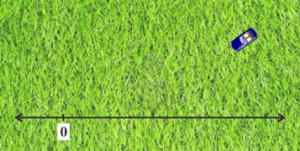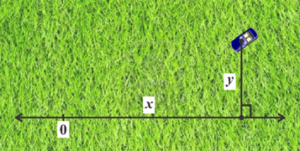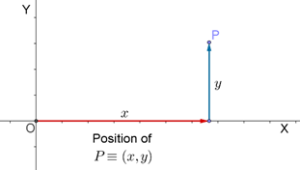# Labeling Points in a Plane

Labeling Points in a Plane

# Labeling Points in a Plane

Now, suppose that the car is moving on a two-dimension plane. Think of a flat field and the car moving about in the field.Someone says that the position of the car at some instant is x = 53.4 m. Does this make sense? The car is not traveling on a (one-dimensional) line. If you are given just one real number as the position of the car, how will you pinpoint its location? You cannot!

Think of this another way. In the figure above, how can you specify the location of the car? Can you specify it using just one real number? Again, the answer is no.

The question then is: how do we label positions in a plane? Let us draw a number line anywhere in the plane, and let the car be positioned at some instant as follows:One way to specify the location of the car with respect to your zero position is as follows: Drop a perpendicular from this position to the number line. Now, specify the distance x along the number line, and then the distance y perpendicular to the number line, which you will have to travel to reach the car from zero:For example, suppose that from zero, one has to travel 52.14 m along the number line, and then 29.87 m perpendicular to the number line, to reach the car. Then, you can say that the position of the car is:

(52.14 m, 29.87 m)

Thus, you have specified the position of the car using two real numbers instead of one.

Let us define more formally our scheme to label positions in a plane. We will use the following approach (as the approach is described, you are urged to make an accurate sketch):

1. Draw a number line X anywhere in the plane and mark zero, the reference point for the entire plane, on it. Call this reference point O.

2. Through this zero, draw another number line Y perpendicular to  X.

3. For any position P in the plane, think of how you will reach P from O, by first traveling along X, and then traveling perpendicular to X (parallel to Y). Note down how much distance you covered traveling along X (call this distance x), and then note how much distance you covered traveling parallel to Y (call this distance y).

4. You can now say that the position of P is (x , y).

Compare your sketch to the following figure:Thus, to label positions in a plane, we need exactly two real numbers.

There’s one interesting point to be noted here. The position you obtain for P will be dependent on how you choose your two number lines X and Y. This should be obvious if you visualize it. If you draw X and Y at different locations than the ones we chose above, then to reach the same position P from your new reference point, you will have to travel different distances along X and parallel to Y. Thus, the label assigned to each point depends on the position of the reference number lines X and Y in the plane.